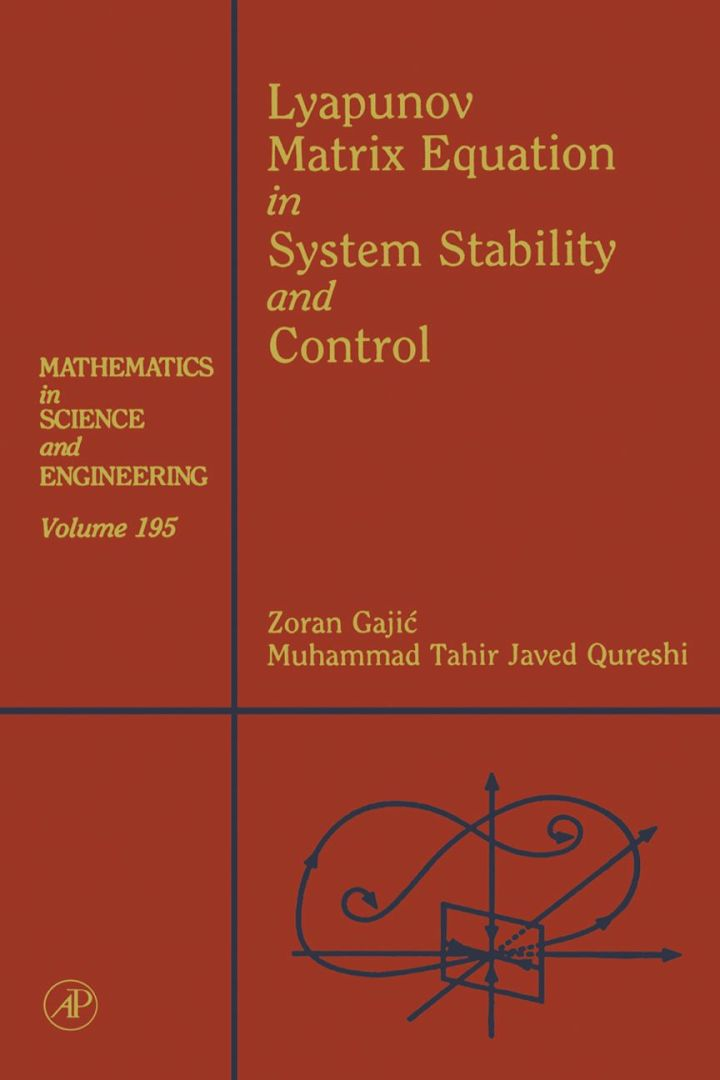# Lyapunov Matrix Equation in System Stability and Control: Mathematics in Science and Engineering V195

## Lyapunov Matrix Equation in System Stability and Control: Mathematics in Science and Engineering V195

By Gajic, Zoran; Qureshi, Muhammad Tahir Javed

### Rent or Buy eTextbook

Expires on Apr 13th, 2022
\$63.95

Publisher List Price: \$64.99
Savings: \$1.04

The Lyapunov and Riccati equations are two of the fundamental equations of control and system theory, having special relevance for system identification, optimization, boundary value problems, power systems, signal processing, and communications.
The Lyapunov Matrix Equation in System Stability and Control covers mathematical developments and applications while providing quick and easy references for solutions to engineering and mathematical problems. Examples of real-world systems are given throughout the text in order to demonstrate the effectiveness of the presented methods and algorithms.
The book will appeal to practicing engineers, theoreticians, applied mathematicians, and graduate students who seek a comprehensive view of the main results of the Lyapunov matrix equation.

Presents techniques for solving and analyzing the algebraic, differential, and difference Lyapunov matrix equations of continuous-time and discrete-time systems
Offers summaries and references at the end of each chapter
Contains examples of the use of the equation to solve real-world problems
Provides quick and easy references for the solutions to engineering and mathematical problems using the Lyapunov equation

Subject: Mathematics & Statistics -> Mathematics -> Mathematics GeneralPublisher: Elsevier S & T 08/1995
Imprint: Academic Press
Language: English
Length: 255 pages

ISBN 10: 0122733703
ISBN 13: 9780122733703
Print ISBN: 9780122733703

Live Chats